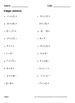Integer Worksheets - addition, subtraction, multiplication and division\$4.00Integer Worksheets.
Integer addition, subtraction, multiplication and division.
Package includes 180 worksheets.

Subtracting integers. (30 Worksheets)
Adding and Subtracting integers. (30 Worksheets)
Multiplying integers. (30 Worksheets)
Dividing integers. (30 Worksheets)
Multiplying and Dividing integers. (30 Worksheets)

----------------------------------

You May Also Like These Task Cards: